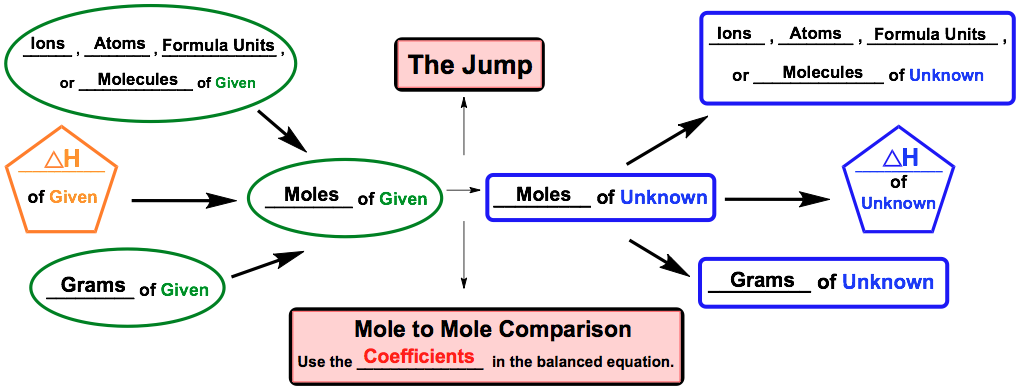Clutch Prep is now a part of Pearson
Ch.7 Energy, Rate and EquilibriumWorksheetSee all chapters

# Thermochemical Equations

See all sections
Sections
Nature of Energy
First Law of Thermodynamics
Endothermic & Exothermic Reactions
Bond Energy
Thermochemical Equations
Heat Capacity
Thermal Equilibrium (Simplified)
Hess's Law
Rate of Reaction
Energy Diagrams
Chemical Equilibrium
The Equilibrium Constant
Le Chatelier's Principle
Solubility Product Constant (Ksp)
Spontaneous Reaction
Entropy (Simplified)
Gibbs Free Energy (Simplified)

Thermochemical Equations involve a balanced chemical equation with a given enthalpy value.

###### Thermochemical Equations

Concept #1: Thermochemical Equations

Concept #2: Thermochemical Stoichiometric Chart

A modified version of the stoichiometric chart can used for thermochemical equations.Example #1: Consider the following thermochemical reaction:

How many grams of MgO are produced during an enthalpy change of –375 kJ?

Practice: Nitromethane (CH3NO2), sometimes used as a fuel for drag racing, burns according to the following reaction:

4 CH3NO2 (l) + 7 O2 (g) → 4 CO2 (g) + 6 H2O (g) + 4 NO2 (g)      ∆Hº = – 2441.6 kJ

How much heat is released by burning 125.0 g of nitromethane (MW: 61.044 g/mol)?

Practice: Consider the following reaction:

2 C6H6 (l) + 15 O2 (g) → 12 CO2 (g) + 6 H2­O (g)   ∆Hº = – 6278 kJ

What volume of benzene (C6H6, d = 0.880 g/mL, molar mass = 78.11 g/mol) is necessary to evolve 5.19 x 109 kJ of heat?

Practice: The creation of liquid methanol is accomplished by the hydrogenation of carbon monoxide:

CO (g) + 2 H2 (g) → CH3OH (l)       ∆Hº = – 128.1 kJ

How much heat (in kJ) is released when 125.0 g CO reacts with 2.32 x 102 g H2?